# Python类与对象最全总结大全（类、实例、属性方法、继承、派生、多态、内建函数）

__init__()“构造器”方法

__new__()“构造器”方法

__del__()“解构器”方法

__init__()应当返回None

staticmethod()和classmethod()内建函数

__bases__类属性

issubclass()

isinstance()

hasattr()、getattr()、setattr()、delattr()

dir()

super()

vars()

# 面向对象编程

## 访问限制

### 私有属性和公有属性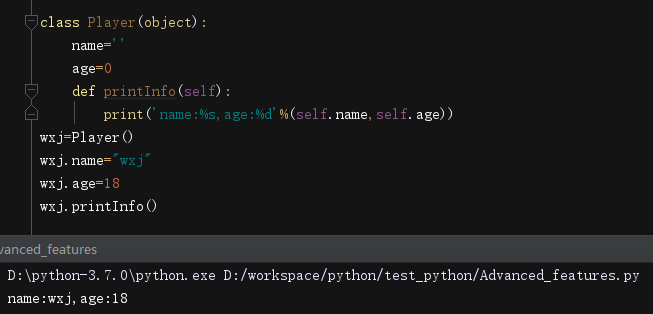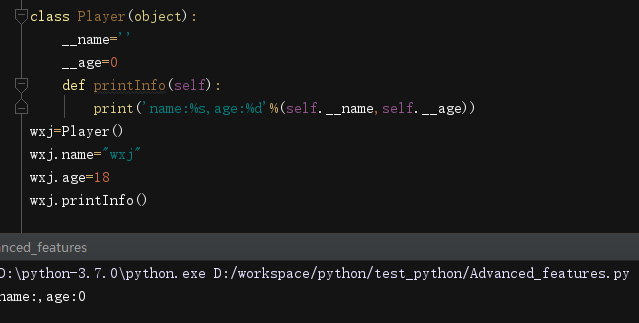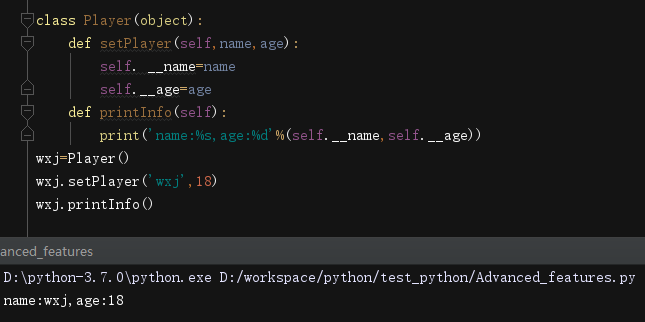### 私有方法的设置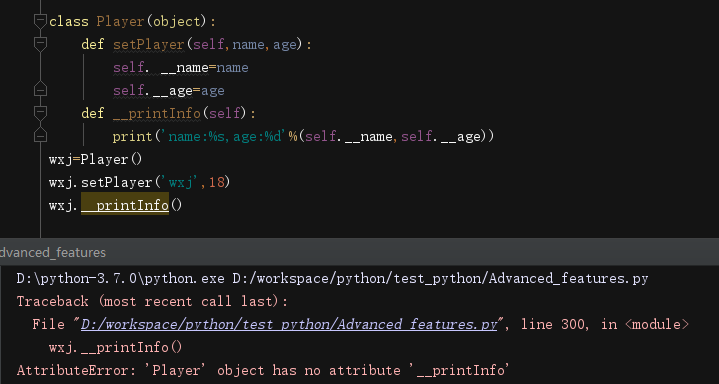## 类

### 创建类

Python使用class关键字来创建。简单的类的声明可以是关键字后紧跟类名：

class className:
'class documentation string' #‘类文档关键字’
pass #类体

## 类属性

1. sys.stdout.write('foo')
2. print myModule myclass.__doc__

### 类的数据属性

class className:
'class documentation string' #‘类文档关键字’
foo=100
print(className.foo)
cn=className()
print(cn.foo)

100

100

### 类的方法属性(函数属性)

1.方法

class MyClass():

def myNoActionMethod(self):

pass

mc=MyClass()

mc.myNoActionMethod()

myNoActionMethod()

Traceback (most recent call last):

File "F:/pythonworkplace/learn_class.py", line 14, in <module>

myNoActionMethod()

NameError: name 'myNoActionMethod' is not defined

MyClass.myNoActionMethod()

Traceback (most recent call last):

File "F:/pythonworkplace/learn_class.py", line 15, in <module>

MyClass.myNoActionMethod()

TypeError: myNoActionMethod() missing 1 required positional argument: 'self'

2.绑定

### 查看类的属性

class MyClass(object):

'MyClass class definition'

myVersion='1.1'

def showMyVersion(self):

print(MyClass.myVersion)

print(dir(MyClass))

print(MyClass.__dict__)

['__class__', '__delattr__', '__dict__', '__dir__', '__doc__', '__eq__', '__format__', '__ge__', '__getattribute__', '__gt__', '__hash__', '__init__', '__init_subclass__', '__le__', '__lt__', '__module__', '__ne__', '__new__', '__reduce__', '__reduce_ex__', '__repr__', '__setattr__', '__sizeof__', '__str__', '__subclasshook__', '__weakref__', 'myVersion', 'showMyVersion']

{'__module__': '__main__', '__doc__': 'MyClass class definition', 'myVersion': '1.1', 'showMyVersion': <function MyClass.showMyVersion at 0x0000018B824B97B8>, '__dict__': <attribute '__dict__' of 'MyClass' objects>, '__weakref__': <attribute '__weakref__' of 'MyClass' objects>}

### 特殊的类属性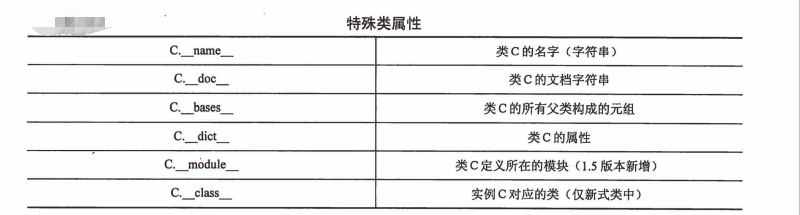print(MyClass.__name__)

print(MyClass.__doc__)

print(MyClass.__bases__)

print(MyClass.__dict__)

print(MyClass.__module__)

print(MyClass.__class__)

MyClass

MyClass class definition

(<class 'object'>,)

{'__module__': '__main__', '__doc__': 'MyClass class definition', 'myVersion': '1.1', 'showMyVersion': <function MyClass.showMyVersion at 0x0000025D441297B8>, '__dict__': <attribute '__dict__' of 'MyClass' objects>, '__weakref__': <attribute '__weakref__' of 'MyClass' objects>}

__main__

<class 'type'>

## 实例

### 初始化：通过调用类对象来创建实例

Python创建实例非常简单，不需要new关键字，直接用过用实例名=类名([参数表])的方式即可，如：

mc=MyClass()

### __init__()“构造器”方法

(个人总结：类似于c++中构造函数，系统默认给你创建，如有需要，可自行创建)

## 实例属性

“实例化”实例属性

### 默认参数提供默认的实例安装

class BoyFriend(object):

def __init__(self,name,age=25,weight=135):

self.name=name

self.age=age

self.weight=weight

def printBoyfriend(self):

print(self.name)

print(self.age)

print(self.weight)

BF=BoyFriend('YB') #对于实例属性中的name在__init__()中是位置参数，不可省略，其他参数是默认参数

BF.printBoyfriend()

abc=BoyFriend('abc',12,36)#对默认参数也做了修改

abc.printBoyfriend()

YB

25

135

abc

12

36

### 实例的属性可在创建实例后自行增减

class C(object):

spam=100

c=C()

c.num=5

c.length=3

print(dir(c))

print(c.__dict__)

['__class__', '__delattr__', '__dict__', '__dir__', '__doc__', '__eq__', '__format__', '__ge__', '__getattribute__', '__gt__', '__hash__', '__init__', '__init_subclass__', '__le__', '__lt__', '__module__', '__ne__', '__new__', '__reduce__', '__reduce_ex__', '__repr__', '__setattr__', '__sizeof__', '__str__', '__subclasshook__', '__weakref__', 'length', 'num', 'spam']

{'num': 5, 'length': 3}

### 查看实例属性

print(dir(BF))

print(BF.__dict__)

['__class__', '__delattr__', '__dict__', '__dir__', '__doc__', '__eq__', '__format__', '__ge__', '__getattribute__', '__gt__', '__hash__', '__init__', '__init_subclass__', '__le__', '__lt__', '__module__', '__ne__', '__new__', '__reduce__', '__reduce_ex__', '__repr__', '__setattr__', '__sizeof__', '__str__', '__subclasshook__', '__weakref__', 'age', 'name', 'printBoyfriend', 'weight']

{'name': 'YB', 'age': 25, 'weight': 135}

### 特殊的实例属性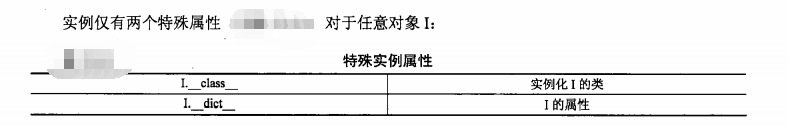### 内建类型属性

x=3+0.14j

print(dir(x))

['__abs__', '__add__', '__bool__', '__class__', '__delattr__', '__dir__', '__divmod__', '__doc__', '__eq__', '__float__', '__floordiv__', '__format__', '__ge__', '__getattribute__', '__getnewargs__', '__gt__', '__hash__', '__init__', '__init_subclass__', '__int__', '__le__', '__lt__', '__mod__', '__mul__', '__ne__', '__neg__', '__new__', '__pos__', '__pow__', '__radd__', '__rdivmod__', '__reduce__', '__reduce_ex__', '__repr__', '__rfloordiv__', '__rmod__', '__rmul__', '__rpow__', '__rsub__', '__rtruediv__', '__setattr__', '__sizeof__', '__str__', '__sub__', '__subclasshook__', '__truediv__', 'conjugate', 'imag', 'real']

## 实例属性VS类属性

### 访问类属性

class C(object):

version=1.2

c=C()

print(C.version)

print(c.version)

C.version+=0.1

print(C.version)

print(c.version)

1.2

1.2

1.3

1.3

### 从实例中访问类属性须谨慎

class C(object):

version=1.2

c=C()

print(C.version)

print(c.version)

c.version+=0.1

print(c.version)

print(C.version)

1.2

1.2

1.3

1.2

class Foo(object):

x={2003:'abc'}

foo=Foo()

print(foo.x)

foo.x='asd'

print(foo.x)

print(Foo.x)

{2003: 'abc'}

{2003: 'abc', 2004: 'asd'}

{2003: 'abc', 2004: 'asd'}

del foo.x #没有遮蔽所以不能删除掉

Traceback (most recent call last):

File "F:/pythonworkplace/learn_class.py", line 65, in <module>

del foo.x #没有遮蔽所以不能删除掉

AttributeError: x

### 类属性持久性

class C(object):

spam=100

c1=C()

print(c1.spam)

C.spam+=100

print(C.spam)

print(c1.spam)

c2=C()

print(c2.spam)

del c1

C.spam+=200

print(c2.spam)

100

200

200

200

400

## 绑定和方法调用

1. 方法仅仅是类内部定义的函数(这意味着方法是类属性而不是实例属性)
2. 方法只有在其所属的类拥有实例时，才能被调用。当存在一个实例时，方法才被认为是绑定到那个实例了。没有实例时方法就是未绑定的。
3. 任何一个方法定义中的第一个参数都是变量self，他表示调用此方法的实例对象。

### 调用非绑定方法

class human():
def __init__(self,id):
self.id=id
class BoyFriend(human):
def __init__(self,id,name):
human.__init__(self,id)  #调用非绑定方法
self.name=name

## 静态方法和类方法

### staticmethod()和classmethod()内建函数

class TestStaticMethod():
def foo():
print('TestStaticMethod')
foo=staticmethod(foo)
class TestClassMethod():
def foo(cls):
print("TestClassMethod")
print('foo() is part of class:',cls.__name__)
foo=classmethod(foo)

tsm=TestStaticMethod()
TestStaticMethod.foo()
tsm.foo()
tcm=TestClassMethod()
TestClassMethod.foo()
tcm.foo()

TestStaticMethod

TestStaticMethod

TestClassMethod

foo() is part of class: TestClassMethod

TestClassMethod

foo() is part of class: TestClassMethod

### 使用函数修饰符

class TestStaticMethod():
@staticmethod
def foo():
print('TestStaticMethod')
class TestClassMethod():
@classmethod
def foo(cls):
print("TestClassMethod")
print('foo() is part of class:',cls.__name__)

## 组合

class NewAddrBookEntry(object):
def __init__(self,nm,ph):
self.name=Name(nm) #创建Name实例
self.phone=Phone(ph)#创建Phone实例
print('Created instance for:',self.name)

## 子类和派生

OOP(面向对象编程)的更强大功能之一是能够使用一个已经定义好的类，扩展它或者对其进行修改，而不会影响系统中使用现存类的其他代码片段。OOD(面向对象设计)允许类特征在子孙类或子类中进行继承。这些子类从基类(或称祖先类、超类)继承它们的核心属性。而且，这些派生可能会扩展到多代。在一个层次的派生关系中的相关类(或者是在类树图中垂直相邻)是父类和子类关系。从同一个父类派生出来的这些类(或者是在类树图中水平相邻)是同胞关系。父类和所有高层类都被认为是祖先。

class Parent(object):#定义父类
def parentMethod(self):
print('parentMethod')
class Child(Parent):#定义子类
def childMethod(self):
print('childMethod')
p=Parent()#父类的实例
p.parentMethod()
c=Child()#子类的实例
c.childMethod()#子类调用它的方法
c.parentMethod()#子类调用父类的方法

parentMethod

childMethod

parentMethod

## 继承

1. 在Python中是支持多重继承的，也就是我们可以为一个类同时指定多个父类
2. 可以在类名的()后边添加多个类，来实现多重继承
3. 多重继承，会使子类同时拥有多个父类，并且会获取到所有父类中的方法
4. 在开发中没有特殊的情况，应该尽量避免使用多重继承，因为多重继承会让我们的代码过于复杂
5. 如果多个父类中有同名的方法，则会现在第一个父类中寻找，然后找第二个，然后找第三个。。。
6. 前边父类的方法会覆盖后边父类的方法

class P(object):
pass
class  C(P):
pass
c=C()
print(c.__class__) #子类“是一个”父类
print(C.__bases__)#子类的父类

<class '__main__.C'>

(<class '__main__.P'>,)

class P(object):
'P class'
def __init__(self):
print('create a instance of',self.__class__.__name__)
class  C(P):
pass

p=P()
print(p.__class__) #显示p所属的类名
print(P.__bases__)#父类的父类
print(P.__doc__)#父类的文档字符串

create a instance of P

<class '__main__.P'>

(<class 'object'>,)

P class

“created an instance”是由__init__()直接输出的。我们也可显示更多关于父类的信息。我们现在来实例化C，展示__init__()(构造)方法在执行过程中是如何继承的:

c=C()
print(c.__class__)
print(C.__bases__)
print(C.__doc__)

create a instance of C

<class '__main__.C'>

(<class '__main__.P'>,)

None

C没有声明 __init__()方法，然而在类C的实例c被创建时，还是会有输出信息。原因在于C继承了P的__init__()。 __base__元组列出了其父类P。需要注意的是文档字符串对类，函数/方法，还有模块来说都是唯一的所以特殊属性doc不会从基类中继承过来

### __bases__类属性

class A(object):pass
class B(A):pass
class C(B):pass
class D(C,B):
pass
print(A.__bases__)
print(C.__bases__)
print(D.__bases__)

(<class 'object'>,)

(<class '__main__.B'>,)

(<class '__main__.C'>, <class '__main__.B'>)

class A(object):pass
class B(A):pass
class C(B):pass
class D(B,C):
pass
print(A.__bases__)
print(C.__bases__)
print(D.__bases__)

Traceback (most recent call last):

File "F:/pythonworkplace/learn_class.py", line 148, in <module>

class D(B,C):

TypeError: Cannot create a consistent method resolution

order (MRO) for bases B, C

### 通过继承覆盖方法

class P(object):
def foo(self):
print("P")
p=P()
p.foo()
class C(P):
def foo(self):
print('C')
c=C()
c.foo()

P

C

class P(object):
def foo(self):
print("P")
class C(P):
def foo(self):
P.foo(self)
print('C')
c=C()
c.foo()

P

C

class P(object):
def foo(self):
print("P")
class C(P):
def foo(self):
super(C,self).foo()
print('C')
c=C()
c.foo()

P

C

### 从标准类型派生

1.不可变类型的例子

class RoundFloat(float):
def __new__(cls,val):
return float.__new__(cls,round(val,2))
print(RoundFloat(1.5656))

class RoundFloat(float):
def __new__(cls,val):
return super(RoundFloat,cls).__new__(cls,round(val,2))
print(RoundFloat(1.5689))

2.可变类型的例子

class SortedKeyDict(dict):
def keys(self):
return sorted(super(SortedKeyDict,self).keys())
d=SortedKeyDict((('zheng-cai',67),('hui-jun',68),('xin-yi',2)))
print('By iterator:',[key for key in d])
print('By keys():',d.keys())

By iterator: ['zheng-cai', 'hui-jun', 'xin-yi']

By keys(): ['hui-jun', 'xin-yi', 'zheng-cai']

### 多重继承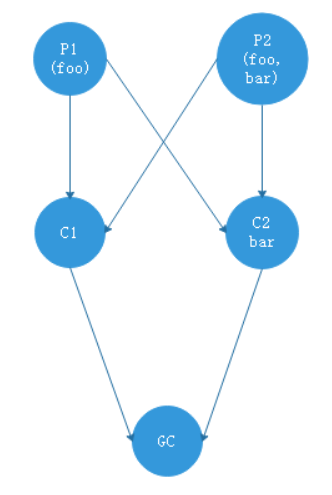class P1(object):
def foo(self):
print('P1-foo')
class P2(object):
def foo(self):
print('P2-foo')
def bar(self):
print('P2-bar')
class C1(P1,P2):
pass
class C2(P1,P2):
def bar(self):
print('C2-bar')
class GC(C1,C2):
pass

gc=GC()
gc.foo()
gc.bar()
print(GC.__mro__)

P1-foo

C2-bar

(<class '__main__.GC'>, <class '__main__.C1'>, <class '__main__.C2'>, <class '__main__.P1'>, <class '__main__.P2'>, <class 'object'>)

z在执行gc.bar()的时候，查找顺序是： GC=> C1 => C2

## 类、实例和其他对象的内建函数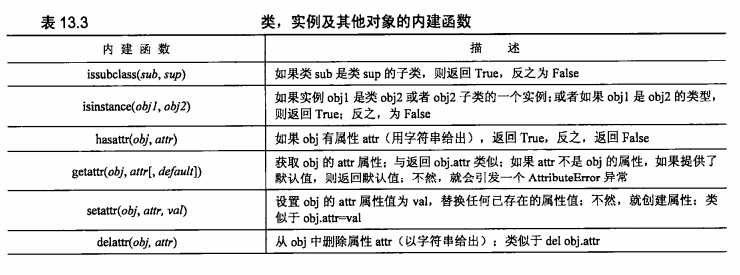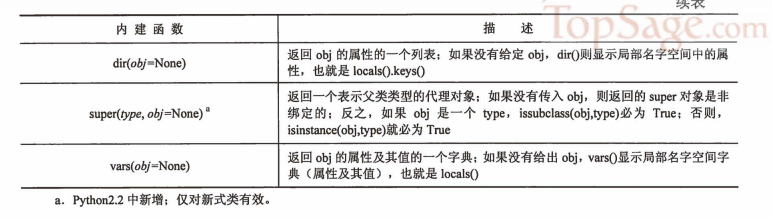### issubclass()

issubclass(sub,sup)

issubclass()布尔函数判定一个类是否是另一个给定类的实例。

issubclass()返回True的情况：给出的子类sub确实是父类sup的一个子类(反之，则为 False)。这个函数也允许“不严格”的子类，即一个类可视为其自身的子类，所以，这个函数如果当sub就是sup(是其本身)，或者从sup派生而来(是其后代)，则返回True。

issubclass()的第二个参数可以是可能的父类组成的元组(tuple)，只要第一个参数是给定元组中任何一个候选类的子类时，就会返回True。

### isinstance()

isinstance()判定一个对象是否是一个给定类的实例。

isinstance(obj1,obj2)

class male():
pass
class female():
pass
m=male()
f=female()
print(isinstance(m,male))
print(isinstance(f,female))
print(isinstance(m,female))

True

True

False

print(isinstance(m,f))

Traceback (most recent call last):

File "F:/pythonworkplace/learn_class.py", line 214, in <module>

print(isinstance(m,f))

TypeError: isinstance() arg 2 must be a type or tuple of types

print(isinstance(2,int))
print(isinstance('123',str))

True

True

### hasattr()、getattr()、setattr()、delattr()

*attr系列函数可以在各种对象下工作，不限于类(class)和实例(instances)。然而，因为在类和实例中使用极其频繁，就在这里列出来了。需要说明的是，当使用这些函数时，你传入你正在处理的对象作为第一个参数，但属性名，也就是这些函数的第二个参数，是这些属性的字符串名字。换句话说在操作obj.attr时，就相当于调用*attr(obj,’attr’…)系列函数——下面的例子讲得很清楚。

hasattr()函数是布尔型的，它的目的就是为了决定一个对象是否有一个特定的属性，一般用于访问某属性前先作一下检查。

getattr()和setattr()函数相应地取得和赋值给对象的属性， getattr()会在你试图读取一个不存在的属性时，引发 AttributeError异常，除非给出那个可选的默认参数。 setattr()将要么加入一个新的属性，要么取代一个已存在的属性。而delattr()函数会从一个对象中删除属性。

class myClass(object):
def __init__(self):
self.foo=100
myInst=myClass()
print(hasattr(myInst,'foo'))#检查myInst对象是否有foo属性
print(getattr(myInst,'foo'))
print(hasattr(myInst,'bar'))

True

100

False

print(getattr(myInst,'bar'))

Traceback (most recent call last):

File "F:/pythonworkplace/learn_class.py", line 226, in <module>

print(getattr(myInst,'bar'))

AttributeError: 'myClass' object has no attribute 'bar'

print(getattr(myInst,'bar','oops!'))#给出可选的默认参数

oops!

setattr(myInst,'bar','my attr')
print(dir(myInst))
print(getattr(myInst,'bar'))
delattr(myInst,'foo')
print(dir(myInst))
print(hasattr(myInst,'foo'))

['__class__', '__delattr__', '__dict__', '__dir__', '__doc__', '__eq__', '__format__', '__ge__', '__getattribute__', '__gt__', '__hash__', '__init__', '__init_subclass__', '__le__', '__lt__', '__module__', '__ne__', '__new__', '__reduce__', '__reduce_ex__', '__repr__', '__setattr__', '__sizeof__', '__str__', '__subclasshook__', '__weakref__', 'bar', 'foo']

my attr

['__class__', '__delattr__', '__dict__', '__dir__', '__doc__', '__eq__', '__format__', '__ge__', '__getattribute__', '__gt__', '__hash__', '__init__', '__init_subclass__', '__le__', '__lt__', '__module__', '__ne__', '__new__', '__reduce__', '__reduce_ex__', '__repr__', '__setattr__', '__sizeof__', '__str__', '__subclasshook__', '__weakref__', 'bar']

False

### dir()

dir()作用在实例上时，显示实例变量，还有在实例所在的类及所有它的基类中定义的方法和类属性。

dir()作用在类上时，则显示类以及它的所有基类的__dict__中的内容。但它不会显示定义在元类中的类属性。

dir()作用在模块上时，则显示模块的__dict__的内容。

dir()不带参数时，则显示调用者的局部变量。

### vars()

vars()内建函数与dir()相似，只是给定的对象参数都必须有一个__dict__属性。

var()返回一个字典，它包含了对象存储于其__dict__中的属性（键）和值。如果提供的对象没有这样一个属性，则会引发一个Type Error异常。如果没有提供对象作为vars()的一个参数，它将显示一个包含本地名字空间的属性（键）及其值的字典，也就是， locals()。

class C(object):
pass
c=C()
c.foo=100
c.bar='Python'
print(c.__dict__)
print(vars(c))

{'foo': 100, 'bar': 'Python'}

{'foo': 100, 'bar': 'Python'}

有问题请下方评论，转载请注明出处，并附有原文链接，谢谢！如有侵权，请及时联系。

08-024091
11-2159
05-091154
03-17472
03-261413
11-29339
03-07217
04-221877
12-15523
11-2034
09-19102
04-18211
11-261951
10-19525
05-27209
06-2616
05-2910
05-07383

### “相关推荐”对你有帮助么？

•非常没帮助
•没帮助
•一般
•有帮助
•非常有帮助WXiujie123456

¥2 ¥4 ¥6 ¥10 ¥20余额支付 (余额：-- )扫码支付获取中扫码支付点击重新获取扫码支付1.余额是钱包充值的虚拟货币，按照1:1的比例进行支付金额的抵扣。
2.余额无法直接购买下载，可以购买VIP、C币套餐、付费专栏及课程。余额充值